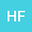loading page

Unification of Fundamental Physics Using Lagrangian-Euclidian Space, Where GR = QFT.
•• Hontas Farmer
Hontas Farmer
College of DuPage
Author Profile## Abstract

Suppose we were to treat the Lagrangians of the theories we wish to unite as coordinates in a space of Lagrangians. In this space the Lagrangians for general relativity (Einstein-Hilbert), the standard model, and the dark sector act as coordinate axes. From here a functional derivative equation is set up for a functional, T, which gives as a result another functional, U, which denotes the Lagrangian for our universe. From there the action $$(\mathscr{S})$$ due to U is computed and shown to converge even at infinite energy. Finally with $$\mathscr{S}$$ a generating functional for all possible interactions is computed and a one inch equation of everything is given$$(Z=e^{i\mathscr{S}})$$. It is the assertion of this paper that with the generating functional detailed herein one may compute any physical quantity of interest including gravitational and dark sector corrections and arrive at a finite result. Specifically graviton-graviton and graviton photon interactions are shown to result in finite observable quantities. It is noted that this is a fully worked out model where GR and QFT are treated on an equal footing by relativizing QFT, or put simply GR=QFT.